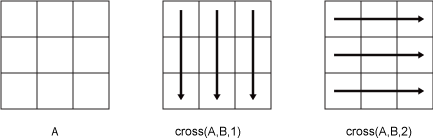# cross

## Syntax

``C = cross(A,B)``
``C = cross(A,B,dim)``

## Description

example

````C = cross(A,B)` returns the cross product of `A` and `B`.If `A` and `B` are vectors, then they must have a length of 3. If `A` and `B` are matrices or multidimensional arrays, then they must have the same size. In this case, the `cross` function treats `A` and `B` as collections of three-element vectors. The function calculates the cross product of corresponding vectors along the first array dimension whose size equals 3.```

example

````C = cross(A,B,dim)` evaluates the cross product of arrays `A` and `B` along dimension, `dim`. `A` and `B` must have the same size, and both `size(A,dim)` and `size(B,dim)` must be 3. The `dim` input is a positive integer scalar.```

## Examples

collapse all

Create two 3-D vectors.

```A = [4 -2 1]; B = [1 -1 3];```

Find the cross product of `A` and `B`. The result, `C`, is a vector that is perpendicular to both `A` and `B`.

`C = cross(A,B)`
```C = 1×3 -5 -11 -2 ```

Use dot products to verify that `C` is perpendicular to `A` and `B`.

`dot(C,A)==0 & dot(C,B)==0`
```ans = logical 1 ```

The result is logical `1` (`true`).

Create two matrices containing random integers.

`A = randi(15,3,5)`
```A = 3×5 13 14 5 15 15 14 10 9 3 8 2 2 15 15 13 ```
`B = randi(25,3,5)`
```B = 3×5 4 20 1 17 10 11 24 22 19 17 23 17 24 19 5 ```

Find the cross product of `A` and `B`.

`C = cross(A,B)`
```C = 3×5 300 122 -114 -228 -181 -291 -198 -105 -30 55 87 136 101 234 175 ```

The result, `C`, contains five independent cross products between the columns of `A` and `B`. For example, `C(:,1)` is equal to the cross product of `A(:,1)` with `B(:,1)`.

Create two 3-by-3-by-3 multidimensional arrays of random integers.

```A = randi(10,3,3,3); B = randi(25,3,3,3);```

Find the cross product of `A` and `B`, treating the rows as vectors.

`C = cross(A,B,2)`
```C = C(:,:,1) = -34 12 62 15 72 -109 -49 8 9 C(:,:,2) = 198 -164 -170 45 0 -18 -55 190 -116 C(:,:,3) = -109 -45 131 1 -74 82 -6 101 -121 ```

The result is a collection of row vectors. For example, `C(1,:,1)` is equal to the cross product of `A(1,:,1)` with `B(1,:,1)`.

Find the cross product of `A` and `B` along the third dimension (`dim = 3`).

`D = cross(A,B,3)`
```D = D(:,:,1) = -14 179 -106 -56 -4 -75 2 -37 10 D(:,:,2) = -37 -162 -37 50 -124 -78 1 63 118 D(:,:,3) = 62 -170 56 46 72 105 -2 -53 -160 ```

The result is a collection of vectors oriented in the third dimension. For example, `D(1,1,:)` is equal to the cross product of `A(1,1,:)` with `B(1,1,:)`.

## Input Arguments

collapse all

Input arrays, specified as numeric arrays.

Data Types: `single` | `double`
Complex Number Support: Yes

Dimension to operate along, specified as a positive integer scalar. The size of dimension `dim` must be 3. If no value is specified, the default is the first array dimension whose size equals 3.

Consider two 2-D input arrays, `A` and `B`:

• `cross(A,B,1)` treats the columns of `A` and `B` as vectors and returns the cross products of corresponding columns.

• `cross(A,B,2)` treats the rows of `A` and `B` as vectors and returns the cross products of corresponding rows.`cross` returns an error if `dim` is greater than `ndims(A)`.

collapse all

### Cross Product

The cross product between two 3-D vectors produces a new vector that is perpendicular to both.

Consider the two vectors

`$\begin{array}{l}A={a}_{1}\stackrel{^}{i}+{a}_{2}\stackrel{^}{j}+{a}_{3}\stackrel{^}{k}\text{\hspace{0.17em}}\text{\hspace{0.17em}},\\ B={b}_{1}\stackrel{^}{i}+{b}_{2}\stackrel{^}{j}+{b}_{3}\stackrel{^}{k}\text{\hspace{0.17em}}\text{\hspace{0.17em}}.\end{array}$`

In terms of a matrix determinant involving the basis vectors $\stackrel{^}{i}$, $\stackrel{^}{j}$, and $\stackrel{^}{k}$, the cross product of A and B is

`$\begin{array}{l}C=A×B=|\begin{array}{cc}\begin{array}{cc}{\stackrel{^}{i}}_{}& {\stackrel{^}{j}}_{}\end{array}& {\stackrel{^}{k}}_{}\\ \begin{array}{cc}\begin{array}{c}{a}_{1}\\ {b}_{1}\end{array}& \begin{array}{c}{a}_{2}\\ {b}_{2}\end{array}\end{array}& \begin{array}{c}{a}_{3}\\ {b}_{3}\end{array}\end{array}|\\ \\ \text{\hspace{0.17em}}\text{\hspace{0.17em}}\text{\hspace{0.17em}}\text{\hspace{0.17em}}\text{\hspace{0.17em}}=\left({a}_{2}{b}_{3}-{a}_{3}{b}_{2}\right)\stackrel{^}{i}+\left({a}_{3}{b}_{1}-{a}_{1}{b}_{3}\right)\stackrel{^}{j}+\left({a}_{1}{b}_{2}-{a}_{2}{b}_{1}\right)\stackrel{^}{k}\text{\hspace{0.17em}}\text{\hspace{0.17em}}.\end{array}$`

Geometrically, $A×B$ is perpendicular to both A and B. The magnitude of the cross product, $‖A×B‖$, is equal to the area of the parallelogram formed using A and B as sides. This area is related to the magnitudes of A and B as well as the angle between the vectors by

`$‖A×B‖=‖A‖\text{\hspace{0.17em}}‖B‖\mathrm{sin}\alpha \text{\hspace{0.17em}}\text{\hspace{0.17em}}.$`

Thus, if A and B are parallel, then the cross product is zero.